Question

# An elevator mass of 2600 kg falls from a height of 25 m after a sudden...

 An elevator mass of 2600 kg falls from a height of 25 m after a sudden failure in the hoisting cable. The mass is stopped by a spring at the bottom of the shaft having a spring constant of 90 kN/m. Determine the spring's compression distance (in m) that brings the falling elevator momentarily to rest.
 An elevator mass of 2600 kg falls from a height of 25 m after a sudden failure in the hoisting cable. The mass is stopped by a spring at the bottom of the shaft having a spring constant of 90 kN/m. Determine the peak magnitude of the acceleration in m/s/s that the elevator undergoes when brought to rest by the spring.
 10p An elevator mass of 2600 kg falls from a height of 25 m after a sudden failure in the hoisting cable. The mass is stopped by a spring at the bottom of the shaft. Determine the spring constant (in kN/m) necessary to bring the elevator and occupants to rest without exceeding an acceleration of 2 g's.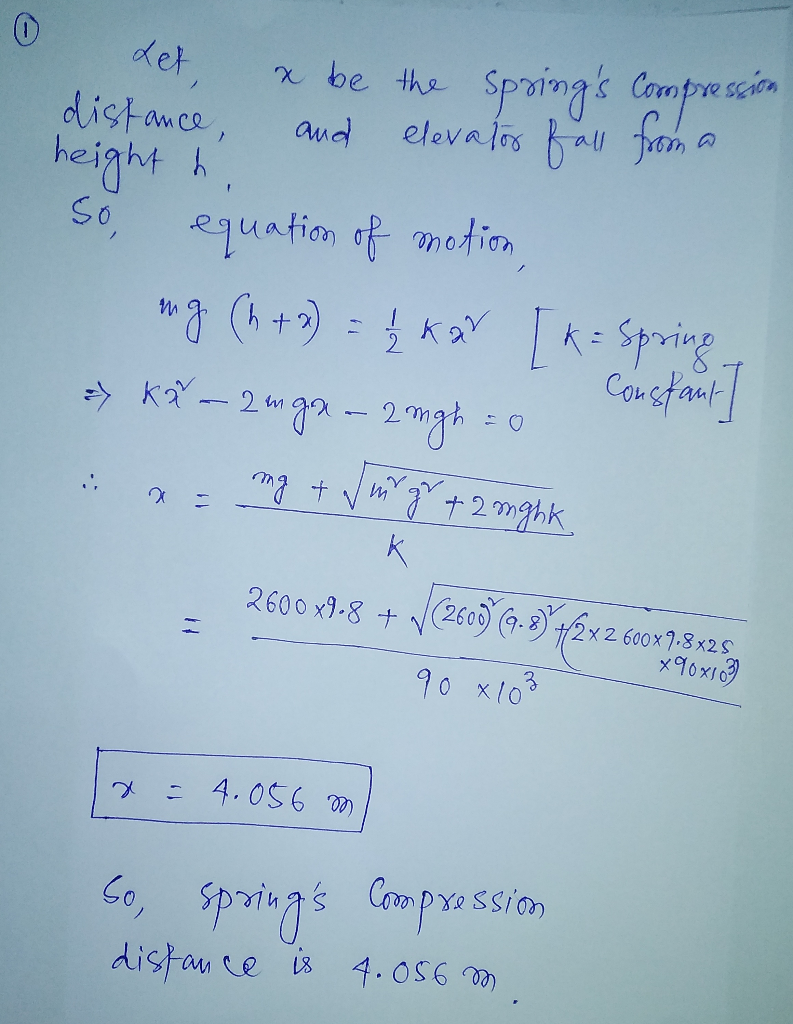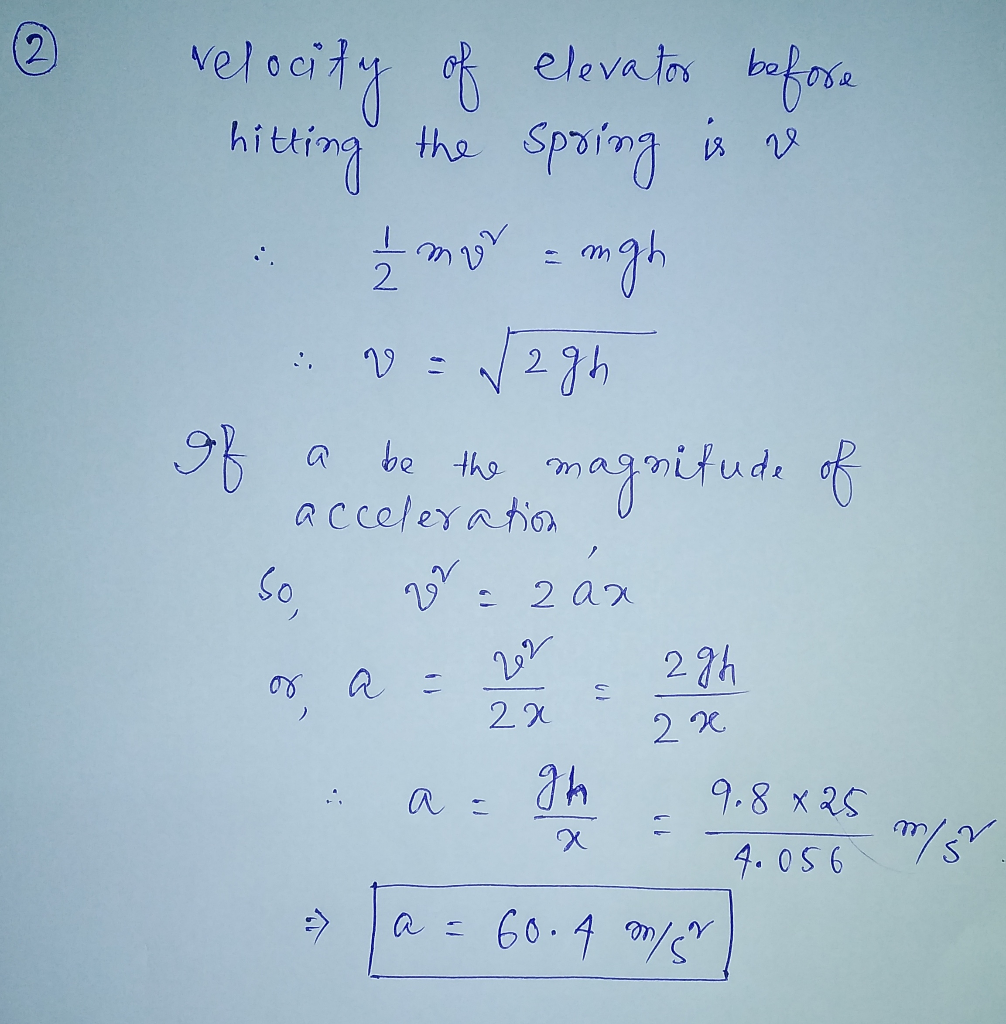With regards. Thanks you.

#### Earn Coins

Coins can be redeemed for fabulous gifts.

Similar Homework Help Questions
• ### An elevator cable breaks and the 1550 kg elevator drops 30 m onto a large spring...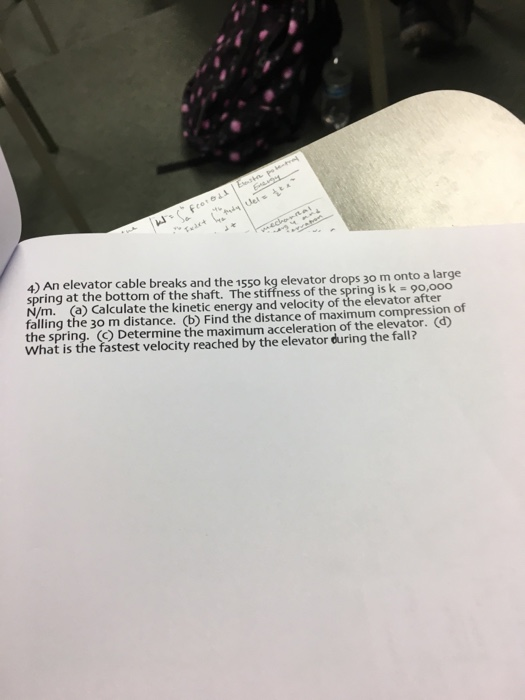An elevator cable breaks and the 1550 kg elevator drops 30 m onto a large spring at the bottom of the shaft. The stiffness of the spring is k = 90, 000 N/m. Calculate the kinetic energy and velocity of the elevator after falling the 30 m distance. Find the distance of maximum compression of the spring. Determine the maximum acceleration of the elevator. What is the fastest velocity reached by the elevator during the fall?

• ### Jumping up before the elevator hits. After the cable snaps and the safety system fails, an...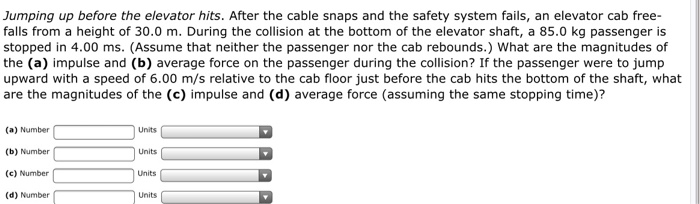Jumping up before the elevator hits. After the cable snaps and the safety system fails, an elevator cab free-falls from a height of 30.0 m. During the collision at the bottom of the elevator shaft, a 85.0 kg passenger is stopped in 4.00 ms. (Assume that neither the passenger nor the cab rebounds.) What are the magnitudes of the (a) impulse and (b) average force on the passenger during the collision? If the passenger were to jump upward with a...

• ### A woman stands on a scale in a moving elevator. Her mass is 57.5 kg, and...

A woman stands on a scale in a moving elevator. Her mass is 57.5 kg, and the combined mass of the elevator and scale is an additional 815 kg. Starting from rest, the elevator accelerates upward. During the acceleration, the hoisting cable applies a force of 9870 N. What does the scale read during the acceleration?

• ### A woman stands on a scale in a moving elevator. Her mass is 62.0 kg, and...

A woman stands on a scale in a moving elevator. Her mass is 62.0 kg, and the combined mass of the elevator and scale is an additional 838 kg. Starting from rest, the elevator accelerates upward. During the acceleration, the hoisting cable applies a force of 9140 N. What does the scale read (in N) during the acceleration?

• ### A woman stands on a scale in a moving elevator. Her mass is 55.0 kg, and...

A woman stands on a scale in a moving elevator. Her mass is 55.0 kg, and the combined mass of the elevator and scale is an additional 797 kg. Starting from rest, the elevator accelerates upward. During the acceleration, the hoisting cable applies a force of 9190 N. What does the scale read (in N) during the acceleration? Number Units

• ### Jumping up before the elevator hits. After the cable snaps and the safety system fails, an...

Jumping up before the elevator hits. After the cable snaps and the safety system fails, an elevator cab free-falls from a height of 37.0 m. During the collision at the bottom of the elevator shaft, a 92.0 kg passenger is stopped in 3.00 ms. (Assume that neither the passenger nor the cab rebounds.) What are the magnitudes of the (a) impulse and (b) average force on the passenger during the collision? If the passenger were to jump upward with a...

• ### A single mass m1 = 3.5 kg hangs from a spring in a motionless elevator. The...

A single mass m1 = 3.5 kg hangs from a spring in a motionless elevator. The spring is extended x = 15 cm from its unstretched length. 1) What is the spring constant of the spring? Now, three masses m1 = 3.5 kg, m2 = 10.5 kg and m3 = 7 kg hang from three identical springs in a motionless elevator. The springs all have the same spring constant that you just calculated above. What is the force the top...

• ### A single mass (m1 = 4.4 kg) hangs from a spring in a motionless elevator.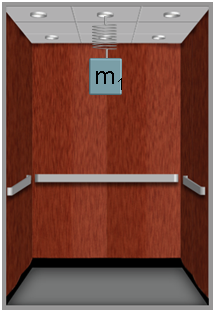A single mass (m1 = 4.4 kg) hangs from a spring in a motionless elevator. The spring constant is k = 270 N/m. 1) What is the distance the spring is stretched from its unstretched length?  2) Now, three masses (m1 = 4.4 kg, m2 = 13.2 kg and m3 = 8.8) hang from three identical springs in a motionless elevator. The springs all have the same spring constant given above. What is the magnitude of the force the bottom spring exerts on the...

• ### A mass m1=5.0 kg hangs suspended by a rope from an elevator ceiling. A second mass...

A mass m1=5.0 kg hangs suspended by a rope from an elevator ceiling. A second mass m2=5.0 kg hangs suspended by a rope from the bottom of mass m1. Starting from rest, the elevator ascends, attaining its maximum speed of 1.8 m/s in 0.80 s. The elevator travels with this constant speed for 5.0 s, undergoes a uniform negative acceleration for 1.5 s, and then comes to rest. Draw a FBD for each mass Find the tension in each rope...

• ### An elevator carries a group of people from the ground floor to the third floor of...

An elevator carries a group of people from the ground floor to the third floor of a building. The elevator and passengers have a combined mass of 2600 kg. The height of one floor is 4.5 m. If the elevator moves at a constant speed and goes from the ground floor to the third floor in one minute, calculate the work done on the elevator by gravity, the work done on the elevator by the cable, the kinetic energy of...